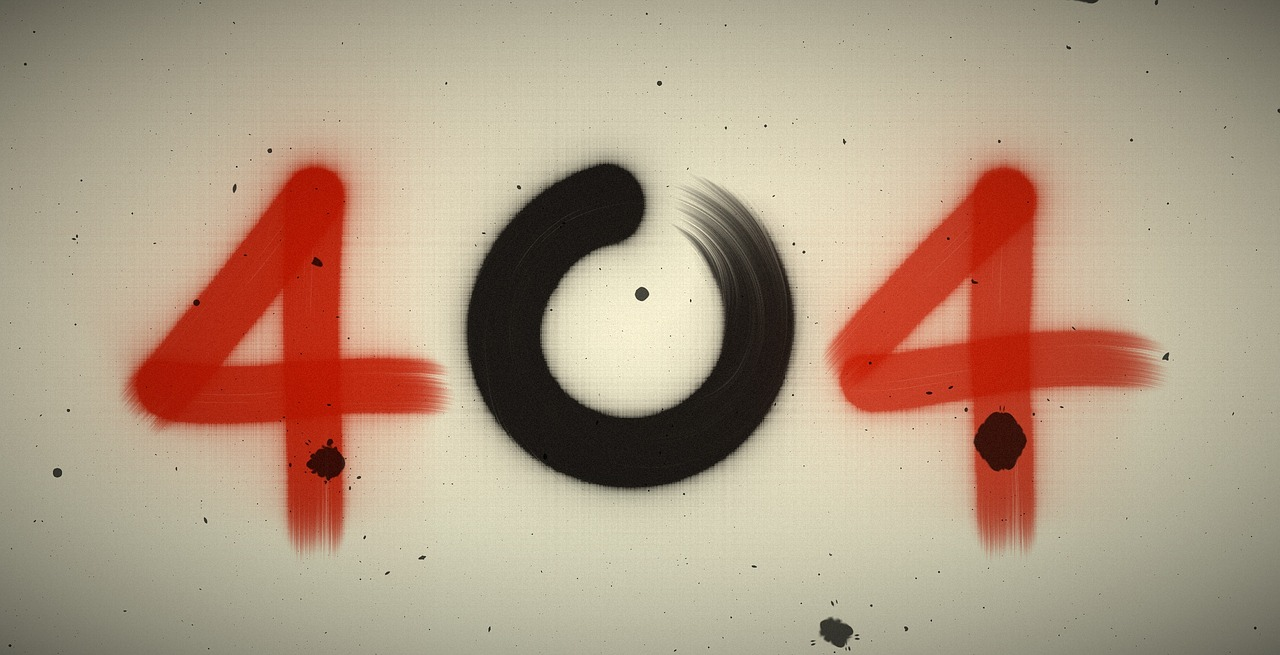"); });

## Post per Page

If you have issues with math, it’s not your fault. The problem is that nobody taught you the most useful math tricks in school. Bright Side put together the best tricks that will help you count easily and quickly. With these, any kind of calculation will be easy as pie.
The easy percent of a number

Quick square calculation

This trick will help you find the square root of a two-digit number that ends with a five. Multiply the first digit by itself and add 1 to it. Then write 25. That’s it!

Quick multiplication by 5

Divide a given number by 2. If the result is a whole number, add 0 at the end. If the result is not a whole number, ignore the decimal and just add 5 at the end.
Easy multiplication by 11

Simple multiplication by 4

The trick here is to multiply your number by 2, and then multiply it by 2 again.
A simply way to calculate tips

If you need to leave 15% of your order as tips, there is a simple way to find how much you need to give. First, find 10% of your sum (divide your sum by 10), and then add another half of the result. That’s your tip.

If you need to multiply large numbers while one of them is even, you can simply regroup them to get an answer.